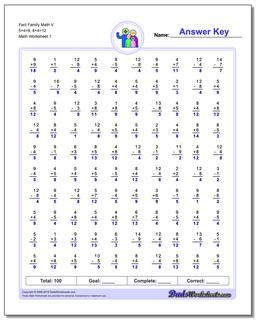# Math Worksheets: Fact Family Math: Fact Family Math: Fact Family Math V 5+4=9, 8+4=12## Fact Family Math V 5+4=9, 8+4=12

PropertyValue
DescriptionFact Family Math V 5+4=9, 8+4=12: These math worksheets have 100 addition and subtraction fact family problems and make for a challenging two minute test.
Resource TypeWorksheet